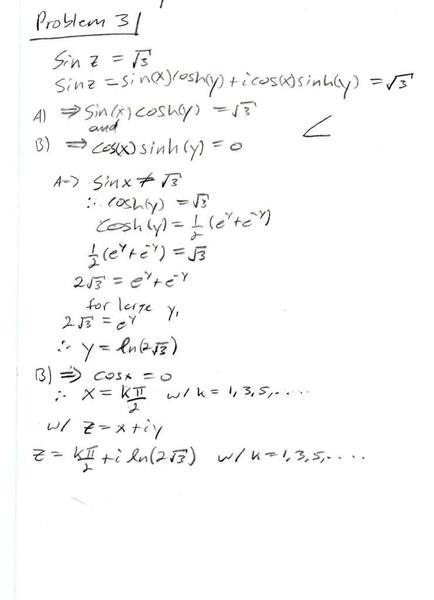# Solving a complex trigonometric equation

Hi, thank you for looking.

## Homework Statement

Solve the following complex equation and carefully plot at least 3 solutions for each. State ALL solutions to each! State exact answers!
Sin(z)=√(3)

## The Attempt at a Solution

Here is my work.

I was a little confused how to tell what function from each case (A or B) I should set equal to solve for the variable. I found sin(x) ≠ √(3) so went with cosh(y)=√(3)

Since I had an answer for y I went with cos(x) = 0 for the next instead of sinh(y) = 0.

Is this a reasonable process?Last edited:

LCKurtz
Homework Helper
Gold Member
You have sin(x)cosh(y) = $\sqrt 3$. While it is true enough that
$\sin(x) \ne \sqrt 3$ why do you conclude that cosh(y) = $\sqrt 3$? You need to re-think that step and keep in mind that this is a simultaneous system of equations you are solving.

You have sin(x)cosh(y) = $\sqrt 3$. While it is true enough that
$\sin(x) \ne \sqrt 3$ why do you conclude that cosh(y) = $\sqrt 3$? You need to re-think that step and keep in mind that this is a simultaneous system of equations you are solving.

Well I thought it was an odd step as well but it was similar to an example the professor did on the board..
I guess I don't know how else to approach the problem from there. You can't solve for/sub x or y between the imaginary and real parts can you?

LCKurtz
Homework Helper
Gold Member
Remember the x and y values you get must satisfy both equations. Since you can figure out what x and y can satisfy your second equation, that limits what you can try in the first equation.

Ok, well sinh(y)=0 therefore y=0.

But if y=0, then cosh(y)=1 and would require sin(x)=√(3)

LCKurtz
Homework Helper
Gold Member
Ok, well sinh(y)=0 therefore y=0.

But if y=0, then cosh(y)=1 and would require sin(x)=√(3)

Right, so that doesn't work. There are two things that make equation B work and you have just ruled out one for equation A. What about the other one?

Sinh(y)=0 only at y=0.

So cos(x)=0 at $\frac{k\pi}{2}$ with k=1,3,5,...
But then its the same as the original assumption I made in A.
Because if I sub $\frac{\pi}{2}$ for x in sin(x) I get 1. or -1 for k=3,7,11

Last edited:
LCKurtz
Homework Helper
Gold Member
Sinh(y)=0 only at y=0.

So cos(x)=0 at $\frac{k\pi}{2}$ with k=1,3,5,...
But then its the same as the original assumption I made in A.
Because if I sub $\frac{\pi}{2}$ for x in sin(x) I get 1. or -1 for k=3,7,11

Yes. So now you are getting close to understanding and giving me the answer to my original question in first post. You have shown that B is satisfied if either sinh(y) = 0 or cos(x) = 0. So those are the only candidates for A. Then you showed y = 0 doesn't lead anywhere. So now you have the only possible values for x, and hence for sin(x). Since sin(x) can be ±1, that both explains why you set that equation $=\sqrt 3$ in the first place and corrects it to using $\pm\sqrt 3$.

Also, another notation thing you might like is to write the values for x like this:

$$x = \frac \pi 2 + k\pi,\ k \hbox{ integer.}$$
to avoid the every other integer thing.

Yes. So now you are getting close to understanding and giving me the answer to my original question in first post. You have shown that B is satisfied if either sinh(y) = 0 or cos(x) = 0. So those are the only candidates for A. Then you showed y = 0 doesn't lead anywhere. So now you have the only possible values for x, and hence for sin(x). Since sin(x) can be ±1, that both explains why you set that equation $=\sqrt 3$ in the first place and corrects it to using $\pm\sqrt 3$.

Also, another notation thing you might like is to write the values for x like this:

$$x = \frac \pi 2 + k\pi,\ k \hbox{ integer.}$$
to avoid the every other integer thing.

Ahh thank you.. It's clicking now.

So when I go about graphing this,
It will be a set of points spread apart by $\frac{\pi}{2}+k\pi$ w/ k= int. at y=ln[2√(3)]

Thank you again for your help.

Last edited: